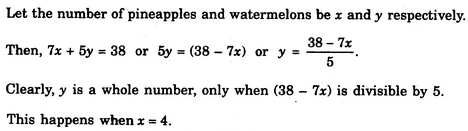Verbal Reasoning - Arithmetic Reasoning - Discussion

Discussion :: Arithmetic Reasoning - Section 1 (Q.No.6)

6.

A pineapple costs Rs. 7 each. A watermelon costs Rs. 5 each. X spends Rs. 38 on these fruits. The number of pineapples purchased is

 [A]. 2 [B]. 3 [C]. 4 [D]. Data inadequate

Explanation:Harshita said: (Jan 19, 2012) Anyone can explain it properly..............

 Surya said: (Mar 22, 2012) I can explain it properly. The total spend amount is 38. So clearly shows here when purchase 4 pineapples at the rate. Rs 7 we get total amount 28. Purchase 2 watermelons at the rate rs 5 we get total amount rs 10. So 28+10=38. You cannot purchase 3 pineapples. So 3*7=21/ 38-21=17. Rs17 it is not sufficient amount for purchase of watermelons.

 Pardhu said: (Oct 22, 2014) If you think logically. Watermelon each one price is Rs == 5 (always round figure like 5*2 = 10, 5*3 = 15). Total spent amount = 38 Rs. Last digit of the total amount is 8 (above 5 table is not matching). By observation like (7*2 = 14, 7*3 = 21, 7*4 = 28). That means 7*4+5*2 = 38 In this manner explanation is length. Just thinking is short.

 Arya said: (Nov 14, 2014) We know the pineapples cost 7 rs each and watermelon 5 rs. Now the normal approach would be to form a quadratic equation. 7x + 5y = 38. Since we have two unknowns and one equation, we need to solve this by TRIAL AND ERROR. Substitute the value of x with every option. For example when x = 2. 14 + 5y = 38. 5y = 24. y = 24/5 which is a decimal number and hence x = 2 can't be a solution. Same case for x = 3. The only option that satisfies the equation x = 4 which gives why = 2. Hence C is the correct answer.

 Anonymous said: (Dec 2, 2014) If x is for pineapple, and y is for watermelon. Then, 7x+5y = 38. we are asked to get number of pineapples, which is x and not y. Hence, x = (38-5y)/7. To achieve a whole number value for pineapple, 38-5y must be divisible by 7. i.e If 38-5y = 28; y = 2; and x = ? No of pineapples = 4

 M Dharma Raju said: (Dec 7, 2014) "x" spent for pineapple and watermelon = Rs.38. Cost of pineapple = Rs.7. Cost of watermelon = Rs.5. The question is how many pineapples you can purchase ? Firstly multiply 38 by 7. The remainder will be 10. According to the question we can purchase 4 pineapples and the balance amount rs 10. We will purchase 02 no of watermelon clearly. This is by with out handling the pen only by mind and quickly answer.

 Ishan Gupta said: (Sep 7, 2015) I can do this by the method of linear equations in two variable.

 Bob said: (Feb 19, 2016) What does Rs stand for?

 Dns said: (May 9, 2016) @Bob. Rs - Rupees.

 Niro said: (Jun 29, 2016) 7 + 7 + 7 + 7 = 28. 5 + 5 = 10. 28 + 10 = 38.

 Siba Nahk said: (Aug 2, 2016) Thanks for giving the detailed solution.

 Jothsna said: (Aug 29, 2016) Simple logic: Here pine apple cost = 7. Watermelon = 5. Total = 38. Then 7 * 4 = 28(pineapple) Watermelon = 5 * 2 = 10. Now 28 + 10 = 38. No of pineapples = 7.

 T L Naidu said: (Sep 26, 2016) Simple logic first sees option there asked pineapple so, 4 * 7 = 28, 2 * 5 = 10.

 Pavan Pj said: (Dec 27, 2016) One pineapple 7rs. Watermelon costs Rs. 5. Total 38. See the choose below, if you buy 2 it became 14rs remaining 24 is not multiples of 5, 3 it became 21, remaining 17 is not multiply of 5. Then the answer is c 7 x 4 = 28 and 5 + 5 = 10 total 38.

 Shubham Tiwary said: (Feb 1, 2017) @All. You can't solve like that because you have got two variables and only one equation.

 Tauseef Ahmad said: (Jun 30, 2018) The easiest method is; 7*4=28, 5*2=10, Total 38, So answer is 4.

 Siri said: (Jan 5, 2019) Very clear, Thanks for the answer @Dharma Raju.

 Kuldeep Singh said: (Aug 15, 2019) The easiest method is; 38-07 =31, 38-14=24, 38-21=17, 38-28=10, 38-35=03, Only no.10 divisble on 5;10+28. So, the Correct answer is 4.

 Miro said: (Aug 19, 2019) Perfect, thanks @Niro.